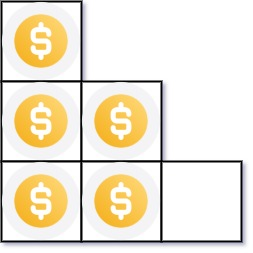# Leetcode 441 - Arranging coins

Note:

• Use math (root = sqrt(8n - 1) - 1 ) / 2.

Question:

You have n coins and you want to build a staircase with these coins. The staircase consists of k rows where the ith row has exactly i coins. The last row of the staircase may be incomplete.

Given the integer n, return the number of complete rows of the staircase you will build.

Example:Code: